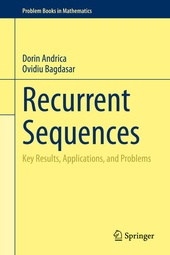# Recurrent Sequences

Key Results, Applications, and Problems

Springer (Verlag)
• erscheint ca. am 20. November 2020

• Buch
• |
• Hardcover
• |
• XV, 407 Seiten
978-3-030-51501-0 (ISBN)

This self-contained text presents state-of-the-art results on recurrent sequences and their applications in algebra, number theory, geometry of the complex plane and discrete mathematics. It is designed to appeal to a wide readership, ranging from scholars and academics, to undergraduate students, or advanced high school and college students training for competitions. The content of the book is very recent, and focuses on areas where significant research is currently taking place. Among the new approaches promoted in this book, the authors highlight the visualization of some recurrences in the complex plane, the concurrent use of algebraic, arithmetic, and trigonometric perspectives on classical number sequences, and links to many applications. It contains techniques which are fundamental in other areas of math and encourages further research on the topic. The introductory chapters only require good understanding of college algebra, complex numbers, analysis and basic combinatorics. For Chapters 3, 4 and 6 the prerequisites include number theory, linear algebra and complex analysis.

The first part of the book presents key theoretical elements required for a good understanding of the topic. The exposition moves on to to fundamental results and key examples of recurrences and their properties. The geometry of linear recurrences in the complex plane is presented in detail through numerous diagrams, which lead to often unexpected connections to combinatorics, number theory, integer sequences, and random number generation. The second part of the book presents a collection of 123 problems with full solutions, illustrating the wide range of topics where recurrent sequences can be found. This material is ideal for consolidating the theoretical knowledge and for preparing students for Olympiads.

 Reihe: Auflage: 1st ed. 2020 Sprache: Englisch Verlagsort: Cham | Schweiz Verlagsgruppe: Springer International Publishing Zielgruppe: Für Beruf und Forschung Illustrationen: 62 | 65 farbige Abbildungen, 2 s/w Abbildungen, 62 farbige Tabellen | 62 Tables, color; 65 Illustrations, color; 2 Illustrations, black and white; XV, 407 p. 67 illus., 65 illus. in color. Maße: Höhe: 23.5 cm | Breite: 15.5 cm Schlagworte: ISBN-13: 978-3-030-51501-0 (9783030515010) DOI: 10.1007/978-3-030-51502-7weitere Ausgaben werden ermittelt

Dorin Andrica is a Professor of Mathematics at the Babe?-Bolyai University of Cluj Napoca, Romania. He has obtained a PhD in Pure Mathematics in 1992 with a thesis on critical point theory with applications to the geometry of differentiable submanifolds. His interests include differential topology (critical point theory with applications, Morse theory with applications), differential geometry, geometry, Lie groups and Lie algebras with applications in geometric mechanics, number theory, discrete mathematics, and mathematics for competitions. Dorin has co-authored Springer textbooks on various topics in mathematics, as well as problem books for olympiad training.

Ovidiu Bagdasar is an Associate Professor in Mathematics at the University of Derby, United Kingdom. He holds PhDs in Applied Mathematics (University of Nottingham, 2011), and Pure Mathematics (Babe?-Bolyai University, 2015), the latter with a thesis entitled "On the geometry and applications of complex recurrent sequences". His research is at the boundary between Mathematics and Computer Science, encompassing areas like number theory, optimization, computational, discrete and applied mathematics. He is the author of the SpringerBriefs volume Concise Computer Mathematics Tutorials on Theory and Problems.
1. Introduction to Recurrence Relations.- 2. Basic Recurrent Sequences.- 3. Arithmetic and Trigonometric Properties of Some Classical Recurrent Sequences.- 4. Generated Functions.- 5. More Second Order Linear Recurrent Sequences.- 6. Higher Order Linear Recurrent Sequences.- 7. Recurrences in Olympiad Training.- 8. Solutions to Proposed Problems.- Appendix A. Complex Geometry anhd Number Theory.- Appendix B.- References.- Index.
This self-contained text presents state-of-the-art results on recurrent sequences and their applications in algebra, number theory, geometry of the complex plane and discrete mathematics. It is designed to appeal to a wide readership, ranging from scholars and academics, to undergraduate students, or advanced high school and college students training for competitions. The content of the book is very recent, and focuses on areas where significant research is currently taking place. Among the new approaches promoted in this book, the authors highlight the visualization of some recurrences in the complex plane, the concurrent use of algebraic, arithmetic, and trigonometric perspectives on classical number sequences, and links to many applications. It contains techniques which are fundamental in other areas of math and encourages further research on the topic. The introductory chapters only require good understanding of college algebra, complex numbers, analysis and basic combinatorics. For Chapters 3, 4 and 6 the prerequisites include number theory, linear algebra and complex analysis.
The first part of the book presents key theoretical elements required for a good understanding of the topic. The exposition moves on to to fundamental results and key examples of recurrences and their properties. The geometry of linear recurrences in the complex plane is presented in detail through numerous diagrams, which lead to often unexpected connections to combinatorics, number theory, integer sequences, and random number generation. The second part of the book presents a collection of 123 problems with full solutions, illustrating the wide range of topics where recurrent sequences can be found. This material is ideal for consolidating the theoretical knowledge and for preparing students for Olympiads.
Schweitzer Klassifikation
• Mathematik
•Algebra
• Mathematik
•Geometrie
• Mathematik
•Zahlentheorie
Thema Klassifikation
Newbooks Subjects & Qualifier
• Mathematik | Informatik
•Mathematik
•Algebra
•Zahlentheorie
• Mathematik | Informatik
•Mathematik
•Geometrie
DNB DDC Sachgruppen
Dewey Decimal Classfication (DDC)
• Science
•Mathematics
•Algebra
• Science
•Mathematics
•General principles of mathematics
• Science
•Mathematics
•Geometry
• Science
•Mathematics
•Mathematics
BIC Classifikation
• Mathematics & science
•Mathematics
•Algebra
• Mathematics & science
•Mathematics
•Discrete mathematics
• Mathematics & science
•Mathematics
•Geometry
• Mathematics & science
•Mathematics
•Number theory
BISAC Classifikation
Warengruppensystematik 2.0
 Ein kurzer Link zu dieser Seite Kopieren Sie diesen Link, um ihn an Dritte weiterzuleiten:als Link merkennach obenDrucken

Noch nicht erschienen

 53,49 € inkl. 5% MwSt.